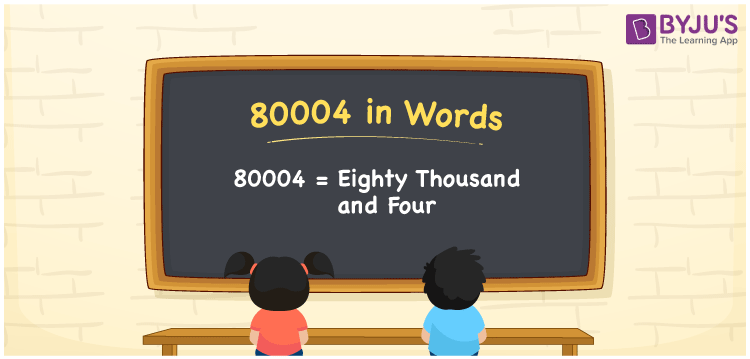# 80004 in Words

We can write 80004 in words as Eighty Thousand and Four. For instance, Riya spent Rs. 80004 for ten days in a 5-star hotel, then you can say, “Riya spent Rupees Eighty Thousand and Four for 10 days in a 5-star hotel”. The number 80004 is a cardinal number since it indicates a specific quantity or value. We can convert any number to word form effortlessly using a place value system. This article explains how to read and write the number 80004 in English using simple tricks.

 80004 in Words Eighty Thousand and Four Eighty Thousand and Four in numerical form 80004

## 80004 in English Words

In Mathematics, with the help of the English alphabet we write the numbers in words. Hence, the number 80004 in English words is written as Eighty Thousand and Four.## How to Write 80004 in Words?

In order to derive the numerical name for 80004, let us create a place value chart of 5 columns as it is a five-digit number.

 Ten Thousands Thousands Hundreds Tens Ones 8 0 0 0 4

Therefore, we can write the expanded form as:

8 x Ten Thousand + 0 x Thousand + 0 x Hundred + 0 x Ten + 4 x One

= 8 x 10000 + 0 x 1000 + 0 x 100 + 0 x 10 + 4 x 1

= 80000 + 0 + 0 + 0 + 4

= 80000 + 4

= 80004

= Eighty Thousand and Four

Hence, 80004 in words is written as Eighty Thousand and Four

Interesting way of writing 80004 in words

8 = Eight

80 = Eighty

800 = Eight Hundred

8000 = Eight Thousand

80004 = Eighty Thousand and Four

Thus, the word form of the number 80004 is Eighty Thousand and Four

80004 is a natural number that precedes 80005 and succeeds 80003

• 80004 in words – Eighty Thousand and Four
• Is 80004 an odd number? – No
• Is 80004 an even number? – Yes
• Is 80004 a perfect square number? – No
• Is 80004 a perfect cube number? – No
• Is 80004 a prime number? – No
• Is 80004 a composite number? – Yes

## Frequently Asked Questions on 80004 in Words

Q1

### How to write 80004 in English words?

80004 in English words is written as Eighty Thousand and Four.
Q2

### Simplify 70000 + 10004, and express in words.

Simplifying 70000 + 10004, we get 80004. Therefore, the number 80004 in words is Eighty Thousand and Four.
Q3

### What is the place value of 4 in 80004?

The place value of 4 in 80004 is Ones.